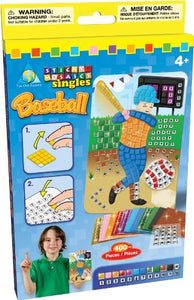# The Orb Factory Sticky Mosaics Sticky Mosaics Singles Baseball

Vendor
The Orb Factory
Regular price
Sold out
Sale price
\$22.33
Quantity must be 1 or more

• T

• h

• e

• O

• r

• b

• F

• a

• c

• t

• o

• r

• y

• S

• t

• i

• c

• k

• y

• M

• o

• s

• a

• i

• c

• s

• S

• t

• i

• c

• k

• y

• M

• o

• s

• a

• i

• c

• s

• S

• i

• n

• g

• l

• e

• s

• B

• a

• s

• e

• b

• a

• l

• l

Its fun to make single sized craft projects with Sticky Mosaics. Kit includes one templated card, one wall hanger and 400+ foam and jewel stickers.